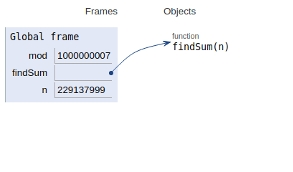# Python Program for Find sum of Series with the n-th term as n^2 â€“ (n-1)^2

## Problem statement

We are given an integer input n and we need to sum of all n terms where the n-th term in a series as expressed below −

Tn = n2 - (n-1)2

We have direct formulas for computing the sum which includes the squared muktiolicaion of n which involves more time complexity . To reduce that we usr modular multiplication approach here

Now let's see the implementation −

## Example

Live Demo

# Python program to find sum of given
# series.
mod = 1000000007
def findSum(n):
return ((n % mod) * (n % mod)) % mod
# main()
n = 229137999
print (findSum(n))

## Output

218194447

All the variables are declared in the global frame as shown in the figure given below −## Conclusion

In this article, we learned about the approach to Find the sum of Series with the n-th term as n^2 – (n-1)^2